# Bayesian inversion using hierarchical prior distributions in the UQlab

Hello,

Here I have a problem regarding how to deal with a hierarchical probabilistic model in the UQlab. Let X ~ Normal( μ ,15) with five (scalar) observations [x1, x2, x3, x4, x5] the prior distribution of μ is μ ~ Normal(50,10), The parameters need to be calibrated is μ .

Given the prior distribution (see attached), how should I encode the forward model in the UQlab? Should I put the probabilistic model “ X ~ Normal( μ ,15)” in the “forward model” or use a “user-defined likelihood” ? if I use the “user-defined likelihood”, should I generate the samples for X to calculate the likelihoods?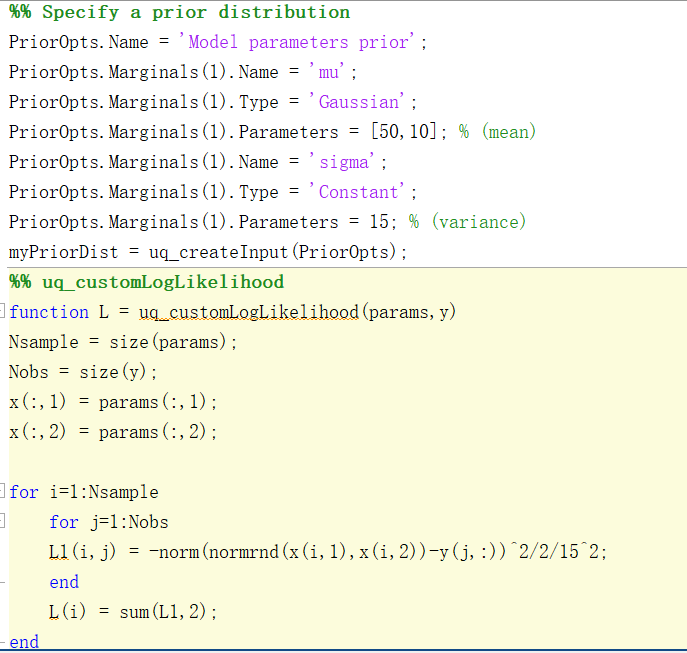Hi @yanglechang and welcome to UQWorld!

So you have a random variable X distributed according to a normal distribution with mean \mu and variance 15, and you want to infer said mean using 5 independent realizations of that random variable \mathcal{X}=\{x_1,\cdots,x_5\}?

This is, indeed, a very simple hierarchical problem as you assign the prior \pi(\mu)=\mathcal{N}(50,10) to the so-called hyperparameter \mu. The Bayesian problem is then written as

\pi(\mu\vert\mathcal{X}) \propto \pi(\mathcal{X}\vert\mu)\pi(\mu).

To solve it, you will need to define a discrepancy model like

\pi(\mathcal{X}\vert\mu) = \prod_{i=1}^5\mathcal{N}(x_i\vert\mu,15).

This can be easily achieved out of the box in the current version of the Bayesian module by specifying the forward model as

\mathcal{M}(\mu) = \mu

and the discrepancy variance as known \sigma^2=15. This should be quite easy!

Let me know, if this solved your problem!

Dear Lechang Yang],

I do not know, if you will need an prior for mu and one for sigma in the end, but I have a small remark to your current code aiming in creating these two priors.

I think that you should use marginals(2) in the definition for sigma and in the following two lines, since otherwise the settings for mu are overwritten and only the prior for sigma is created. I suggest to have a look for a similar situation in the Section PRIOR DISTRIBUTION OF THE MODEL PARAMETERS in the UQLab example INVERSION: SIMPLE BEAM CALIBRATION

Hello,

@paulremo Many thanks for your detailed comments. They are very helpful ! @olaf.klein Thank you for revealing this issue. Yes, it is a mistake. I indeed want to assign two different prior distributions for μ and σ.

Maybe my previous description is little bit confusing but my concern is acctually about how to build a Bayesian inversion model with hierarchical prior distributions in the QUlab.

Let it be more clear, for a deterministic forward model Y = f(X) with observations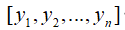, the likelihood is given in the normal distribution form as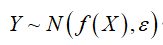. The prior distribution of the input variable X is also a normal distribution X ~ N( μ , σ ), where the unknown parameters μ and σ fall with the intervals [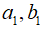] and [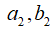] (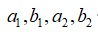are fixed values) repectively. That is μ ~ Uniform (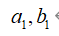) , σ ~ Uniform () . If the parameters need to be calibrated are μ and σ , how to assign their prior distributions in the UQlab ?

In addition, ifare not given in the fixed values but in probabilistic forms, i.e. the prior distributions are in a 3-level hierarchical form, how to build the Bayesian inversion model in this scenario ?

Many thanks for your patience !
Best regards

So the first problem can be solved right out of the box in the inversion module by setting up the problem as follows:

First, define a simple forward model (identity function)

ModelOpts.mString = 'X';
ModelOpts.isVectorized = true;
myForwardModel = uq_createModel(ModelOpts);


store the measurements

myData.y = [y1; y2; y3; ...; yN];
myData.Name = 'Measurements of y';


define a prior distribution on \mu

PriorOpts.Marginals.Name = 'mu';
PriorOpts.Marginals.Type = 'Uniform';
PriorOpts.Marginals.Parameters = [a1 b1];
myPriorDist = uq_createInput(PriorOpts);


supply the discrepancy model

SigmaOpts.Marginals.Name = 'Sigma2';
SigmaOpts.Marginals.Type = 'Uniform';
SigmaOpts.Marginals.Parameters = [a2 b2];

DiscrepancyOpts.Type = 'Gaussian';


and finally, define the object that stores all this information

BayesOpts.Type = 'Inversion';
BayesOpts.Data = myData;
BayesOpts.Discrepancy = DiscrepancyOpts;
BayesOpts.Prior = myPriorDist;


That’s it, now you should be able to run the analysis with

myBayesianAnalysis = uq_createAnalysis(BayesOpts);
% Print out a report of the results:
uq_print(myBayesianAnalysis)
% Create a graphical representation of the results:
uq_display(myBayesianAnalysis)


That should do the trick. Keep in mind that this simple hierarchical problem is a very special case that is supported only because it meets the following conditions (I hope I didn’t forget any):

1. You assume that X\sim\mathcal{N} (normally distributed) which is incidentally the only provided discrepancy model;
2. There are not more than two levels in your problem’s hierarchy;
3. You are inferring information about the parameters using observations of those parameters thus reducing the forward model to the identity function \mathcal{M}(\mu)=\mu;
4. Implicitly you assume perfect, i.e. noise-free, measurements.

If any of those conditions were not met, you would have to use the user-defined likelihood function feature to set up your problem.

Assume now that you assign a prior \pi(\theta) also on the parameters \mathbf{\theta}=(a_1,a_2,b_1,b_2), the 2. condition in the list above is not met because your problem now has three levels of hierarchy and you would have to extend your Bayesian problem definition to

\pi(\mu\vert\mathcal{X})\propto\pi(\mathcal{X}\vert\mu)\pi(\mu\vert\theta)\pi(\theta).

To fit into the framework provided by the Bayesian module in UQLab, your user-defined likelihood function should then return something like

\mathcal{L}(\theta) = \int \pi(\mathcal{X}\vert\mu)\pi(\mu\vert\theta)\,\mathrm{d}\mu,

i.e. marginalize over the hyper parameter \mu to be a function of only the (hyper-)hyperparameters \theta.

I hope this helps!

Hi @paulremo
My Bayesian model is actually a two-level hierarchy, but the available data is [y_1, y_2, … ,y_n], which is about Y but not about X. That is to say, given the forward model Y = f(X) and discrepancy model Y ~ N(f(X), ε^2), the prior distribution \pi(\theta,\sigma) is not directly involved in the forward model. To get the simulated values of Y, samples of (\theta,\sigma) need to be first generated and then generate the samples of X using X ~ N(\mu, \sigma^2) and then pass to Y. In this situation, I just wonder if I have to use a user-defined likelihood function? The Bayesian model in this condition is

\pi(\mu,\sigma|Y)\propto\pi(Y|\mu,\sigma)\pi(\mu,\sigma)

Best regards,
Lechang

Yes, in this situation you will have to use the user-defined likelihood function feature. I am, however, confused by your Bayesian model equation. You said previously that you want to assign a (hyper-)prior to the parameters \theta that define the distribution of \mu and \sigma. Calling the data \mathcal{Y}=\{y_1,\cdots,y_n\}, in this case your Bayesian model should look like this

\pi(\theta\vert\mathcal{Y})\propto\pi(\mathcal{Y}\vert\mu,\sigma)\pi(\mu,\sigma\vert\theta)\pi(\theta)

Similarly to what I mentioned in my previous post, your likelihood function should then return something like

\mathcal{L}(\theta)=\int\int \pi(\mathcal{Y}\vert\mu,\sigma)\pi(\mu,\sigma\vert\theta)\,\mathrm{d}\mu\,\mathrm{d}\sigma,

Is this what you are trying to do?

Hi @paulremo
Thank you for your prompt reply. In my previous post, I wrote

That is, the output variable Y is assumed to follow a normal distribution Y ~ N(\mu_y, ε^2) where Y = f(X) is a deterministic propagation model (forward model) and \varepsilon is the noise. I want to use a normal distribution distribution X ~ N(\mu, \sigma^2) as the prior of the input variable X, but I don’t know the exact values of the parameter \mu and \sigma, so I assign uniform distributions \mu ~ Unif(a_1,b_1) for \mu and \sigma ~ Unif(a_2,b_2) for \sigma since I know their lower and upper bounds. In this situation, the Bayesian model should be written as

\pi(\mu,\sigma|Y)\propto\pi(Y|\mu,\sigma)\pi(\mu,\sigma)

or

\pi(\theta|Y)\propto\pi(Y|\mu,\sigma)\pi(\mu,\sigma|\theta)\pi(\theta), \theta = (a_1,b_1,a_2,b_2) ?

Is there something wrong with my understanding?

Best,
Lechang

No - this looks good to me! If you can, it would be great to share your implementation of the user-defined likelihood function for others to use as well1 Like